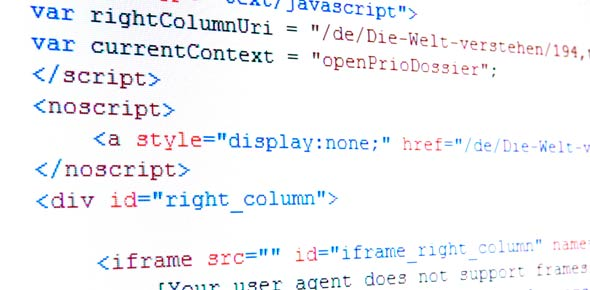Programming Trivia Quiz Questions!

10 Questions | Total Attempts: 79Settings• 1.
In the Invaders.js file there are a total of four functions. Which function moves each invader a step down when it gets to the boundary of the game area?
• A.

• B.

• C.

• D.

• 2.
In the file Main.js, there is a function that creates a new Invader. What is the name of the function?
• A.

Create

• B.

• C.

Instantiate

• D.

Bench

• 3.
Here's a code fragment of a For loop. The For loop increments the variable called i. When the For loop ends what is the final value of i? Here's the code fragment: var i:int = 0; for(i = 0; i < 361; i++){      print("What's the final value of i?"); }
• 4.
In the file bullet.js there is a function there that causes the bullet to detect if it collides with something. When that function does trigger, where would the bullet be positioned? Phrase it like this "120 in z".
• 5.
There is  a function in ship.js that runs when the ship object comes into being. That function runs which line?
• A.

Bullet.transform.position.x = 1000;

• B.

Bullet.Transform.Position.X = 100;

• C.

Bullet.transform.position.x = 100;

• D.

Bullet.transform.position.x = 10;

• 6.
In the file Main.js, there is  an unused function that you can delete. What's the name of the function?
• 7.
• 8.
In the file bullet.js, I have two variables with two different variable types. Which of the following below is not one of them?
• A.

Boolean

• B.

Float

• C.

Int

• D.

String

• 9.
In Invader.js there is a function there called OnTriggerEnter. It accepts a collision object which I have assigned to a variable called col. I can get the name of the collision object by calling this: col.gameObject.name So I'm looking for three collision objects in a series of if statements which are those names?
• A.

LeftBarrier

• B.

Bullet

• C.

RightBarrier

• D.

Bullet

• 10.
Answer this: var x:int = 25; var y:int = 48; if(x + y < 74){      print("That's right."); }else{     print("That's wrong."); }
Related TopicsBack to top